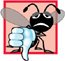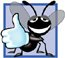# Decision Making: Equality and Relational Operators

### Decision Making Equality and Relational Operators

This section introduces a simple version of C++'s if statement that allows a program to make a decision based on the truth or falsity of some condition. If the condition is met, i.e., the condition is true, the statement in the body of the if statement is executed. If the condition is not met, i.e., the condition is false, the body statement is not executed. We will see an example shortly.

Conditions in if statements can be formed by using the equality operators and relational operators summarized in Fig. 2.12. The relational operators all have the same level of precedence and associate left to right. The equality operators both have the same level of precedence, which is lower than that of the relational operators, and associate left to right.

Figure 2.12. Equality and relational operators.

Standard algebraic equality or relational operator

C++ equality or relational operator

Sample C++ condition

Meaning of C++ condition

Relational operators

>

>

x > y

x is greater than y

<

<

x < y

x is less than y>=

x >= y

x is greater than or equal to y<=

x <= y

x is less than or equal to y

Equality operators

=

==

x == y

x is equal to y!=

x != y

x is not equal to y

Common Programming Error 2.5A syntax error will occur if any of the operators ==, !=, >= and <= appears with spaces between its pair of symbols.

Common Programming Error 2.6Reversing the order of the pair of symbols in any of the operators !=, >= and <= (by writing them as =!, => and =<, respectively) is normally a syntax error. In some cases, writing != as =! will not be a syntax error, but almost certainly will be a logic error that has an effect at execution time. You will understand why when you learn about logical operators in Chapter 5. A fatal logic error causes a program to fail and terminate prematurely. A nonfatal logic error allows a program to continue executing, but usually produces incorrect results.

Common Programming Error 2.7Confusing the equality operator == with the assignment operator = results in logic errors. The equality operator should be read "is equal to," and the assignment operator should be read "gets" or "gets the value of" or "is assigned the value of." Some people prefer to read the equality operator as "double equals." As we discuss in Section 5.9, confusing these operators may not necessarily cause an easy-to-recognize syntax error, but may cause extremely subtle logic errors.

The following example uses six if statements to compare two numbers input by the user. If the condition in any of these if statements is satisfied, the output statement associated with that if statement is executed. Figure 2.13 shows the program and the input/output dialogs of three sample executions.

Figure 2.13. Equality and relational operators.

(This item is displayed on pages 53 - 54 in the print version)

``` 1 // Fig. 2.13: fig02_13.cpp
2 // Comparing integers using if statements, relational operators
3 // and equality operators.
4 #include  // allows program to perform input and output
5
6 using std::cout; // program uses cout
7 using std::cin; // program uses cin
8 using std::endl; // program uses endl
9
10 // function main begins program execution
11 int main()
12 {
13 int number1; // first integer to compare
14 int number2; // second integer to compare
15
16 cout << "Enter two integers to compare: "; // prompt user for data
17 cin >> number1 >> number2; // read two integers from user
18
19 if ( number1 == number2 )
20  cout << number1 << " == " << number2 << endl;
21
22 if ( number1 != number2 )
23 cout << number1 << " != " << number2 << endl;
24
25 if ( number1 < number2 )
26 cout << number1 << " < " << number2 << endl;
27
28 if ( number1 > number2 )
29 cout << number1 << " > " << number2 << endl;
30
31 if ( number1 <= number2 )
32 cout << number1 << " <= " << number2 << endl;
33
34 if ( number1 >= number2 )
35 cout << number1 << " >= " << number2 << endl;
36
37 return 0; // indicate that program ended successfully
38
39 } // end function main
```

 ``` Enter two integers to compare: 3 7 3 != 7 3 < 7 3 <= 7 ```

 ``` Enter two integers to compare: 22 12 22 != 12 22 > 12 22 >= 12 ```

 ``` Enter two integers to compare: 7 7 7 == 7 7 <= 7 7 >= 7 ```

Lines 68

```using std::cout; // program uses cout
using std::cin; // program uses cin
using std::endl; // program uses endl
```

are using declarations that eliminate the need to repeat the std:: prefix as we did in earlier programs. Once we insert these using declarations, we can write cout instead of std::cout, cin instead of std::cin and endl instead of std::endl, respectively, in the remainder of the program. [Note: From this point forward in the book, each example contains one or more using declarations.]

Good Programming Practice 2.15Place using declarations immediately after the #include to which they refer.

Lines 1314

```int number1; // first integer to compare
int number2; // second integer to compare
```

declare the variables used in the program. Remember that variables may be declared in one declaration or in multiple declarations.

The program uses cascaded stream extraction operations (line 17) to input two integers. Remember that we are allowed to write cin (instead of std::cin) because of line 7. First a value is read into variable number1, then a value is read into variable number2.

The if statement at lines 1920

```if ( number1 == number2 )
cout << number1 << " == " << number2 << endl;
```

compares the values of variables number1 and number2 to test for equality. If the values are equal, the statement at line 20 displays a line of text indicating that the numbers are equal. If the conditions are true in one or more of the if statements starting at lines 22, 25, 28, 31 and 34, the corresponding body statement displays an appropriate line of text.

Notice that each if statement in Fig. 2.13 has a single statement in its body and that each body statement is indented. In Chapter 4 we show how to specify if statements with multiple-statement bodies (by enclosing the body statements in a pair of braces, { }, creating what is called a compound statement or a block).

Good Programming Practice 2.16Indent the statement(s) in the body of an if statement to enhance readability.

Good Programming Practice 2.17For readability, there should be no more than one statement per line in a program.

Common Programming Error 2.8Placing a semicolon immediately after the right parenthesis after the condition in an if statement is often a logic error (although not a syntax error). The semicolon causes the body of the if statement to be empty, so the if statement performs no action, regardless of whether or not its condition is true. Worse yet, the original body statement of the if statement now would become a statement in sequence with the if statement and would always execute, often causing the program to produce incorrect results.

Note the use of white space in Fig. 2.13. Recall that white-space characters, such as tabs, newlines and spaces, are normally ignored by the compiler. So, statements may be split over several lines and may be spaced according to the programmer's preferences. It is a syntax error to split identifiers, strings (such as "hello") and constants (such as the number 1000) over several lines.

Common Programming Error 2.9It is a syntax error to split an identifier by inserting white-space characters (e.g., writing main as ma in).

Good Programming Practice 2.18A lengthy statement may be spread over several lines. If a single statement must be split across lines, choose meaningful breaking points, such as after a comma in a comma-separated list, or after an operator in a lengthy expression. If a statement is split across two or more lines, indent all subsequent lines and left-align the group.

Figure 2.14 shows the precedence and associativity of the operators introduced in this chapter. The operators are shown top to bottom in decreasing order of precedence. Notice that all these operators, with the exception of the assignment operator =, associate from left to right. Addition is left-associative, so an expression like x + y + z is evaluated as if it had been written (x + y) + z. The assignment operator = associates from right to left, so an expression such as x = y = 0 is evaluated as if it had been written x = (y = 0), which, as we will soon see, first assigns 0 to y then assigns the result of that assignment0to x.

Figure 2.14. Precedence and associativity of the operators discussed so far.

Operators

Associativity

Type

()

left to right

parentheses

*

/

%

left to right

multiplicative

+

-

left to right

<<

>>

left to right

stream insertion/extraction

<

<=

>

>=

left to right

relational

==

!=

left to right

equality

=

right to left

assignment

Good Programming Practice 2.19Refer to the operator precedence and associativity chart when writing expressions containing many operators. Confirm that the operators in the expression are performed in the order you expect. If you are uncertain about the order of evaluation in a complex expression, break the expression into smaller statements or use parentheses to force the order of evaluation, exactly as you would do in an algebraic expression. Be sure to observe that some operators such as assignment (=) associate right to left rather than left to right.C++ How to Program (5th Edition)
ISBN: 0131857576
EAN: 2147483647
Year: 2004
Pages: 627